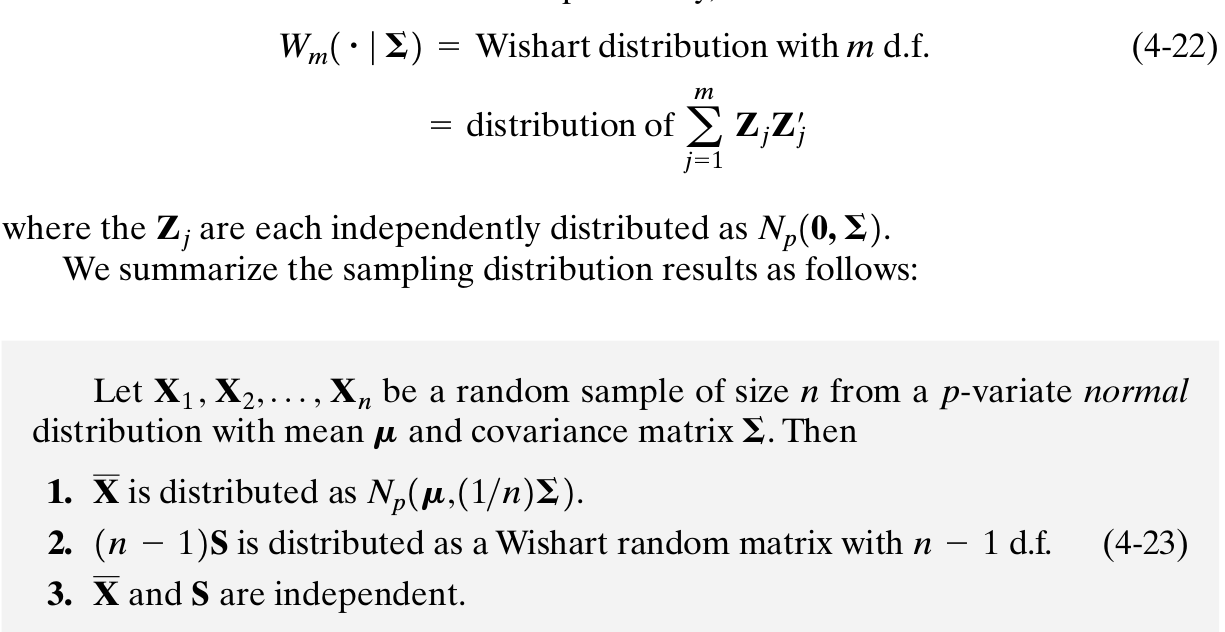# Statistics¶

## Distribution relationships¶

### Gamma & Wishart¶

Gamma(k,\theta) = Wishart(\nu, \sigma^2)\,,

where $k=\nu/2$ and $\theta=2\sigma^2$.

### Gamma & Chi-squared¶

• $\chi^2_k = Gamma(k/2, 2)$
• $c\chi^2_k = Gamma(k/2, 2c)$
• $\sum_{i=1}^n\chi^2_k(\lambda) = \chi^2_{nk}(n\lambda)$.

## Calculus¶

1. $\int_0^\infty e^{-x^2}dx$, see Impossible Integral.
2. Integral Inequality Absolute Value: $|\int^b_af(x)g(x) dx∣\le\int_a^b|f(x)|⋅|g(x)| dx$
3. $\sum \dfrac{1}{n\log n}$ and $\sum \dfrac{1}{n\log n\log(\log n)}$ diverges, while $\sum\dfrac{1}{x\log^2x}$ converges, refer to Infinite series … for more details.
4. $\int_{-\infty}^\infty \sin x/x = \pi$, refer to math.stackexchange

## Simplex Volume¶

A simplex in $n$-dimensional Euclidean space is a convex solid with $n+1$ vertices, its volume is

V_n = \frac{1}{n!}h_nh_{n-1}\cdots h_1\,,

where $h_i$ is the distance between the $i$-th and the $i+1$-th vertices.

See Simplex Volumes and the Cayley-Menger Determinant for more details.

## Interesting Graphs¶

Cardioid (心形)：$r=2a(1+\cos\theta)$

## Confidence set for parameter vector in linear regression¶

Confidence set for parameter vector in linear regression

## Spherical and Elliptical Distributions¶

Spherical and Elliptical Distributions

## Wishart Distribution¶## Subscript notation in expectations.¶

Always confused the risk function

\E_P[L(\theta(P),\hat\theta)]\,,

more clearly one for me is

R_T(P)=\E[L(P,T(X))]=\int_{\cal X}L(P,T(x))dP_X(x)

And one related question asked in Subscript notation in expectations

## Fisher Information matrix and Hessian matrix¶

the second derivative of the log-likelihood evaluated at the maximum likelihood estimates (MLE) is the observed Fisher information. This is exactly what most optimization algorithms like optim in R return: the Hessian evaluated at the MLE. When the negative log-likelihood is minimized, the negative Hessian is returned. As you correctly point out, the estimated standard errors of the MLE are the square roots of the diagonal elements of the inverse of the observed Fisher information matrix. In other words: The square roots of the diagonal elements of the inverse of the Hessian (or the negative Hessian) are the estimated standard errors.

\mathbf{I}(\theta)=-\frac{\partial^{2}}{\partial\theta_{i}\partial\theta_{j}}l(\theta),~~~~ 1\leq i, j\leq p

and

\mathbf{H}(\theta)=\frac{\partial^{2}}{\partial\theta_{i}\partial\theta_{j}}l(\theta),~~~~ 1\leq i, j\leq p

## Prove $\sum_{i=1}^n(y_i-\hat y_i)(\hat y_i-\bar y) = 0$ in linear regression¶

Note that $X'(Y-X\beta) = 0$, then take the first column of $X$, i.e., the first row of $X'$, then we can get $\sum_{i=1}^ny_i=\sum_{i=1}^n\hat y_i$.

Last update: July 12, 2020
Created: December 24, 2018